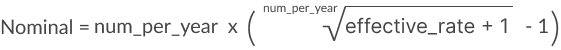# Nominal

The Nominal function returns the nominal annual interest rate.

Lending institutions advertise the nominal rate of their loans. The nominal interest rate does not take into account the effect of compounding. The effective interest rate takes the compounding period into account, and it is a more accurate measure of interest charges.

Nominal is part of the set of financial functions that Sigma supports.

## Syntax

`Nominal(effective_rate, num_per_year)`

The Nominal function syntax has the following arguments:

effective_rate
Required.
Effective interest rate
To use 7%, use the value 0.07.
num_per_year
Required.
Number of compounding periods per year

The general formula for the Nominal function is:## Examples

`Nominal(.07, 12)`

The nominal annual interest rate for an effective rate of 7%, paid 12 times a year (monthly). This example returns 6.78%.

`Nominal(0.7, 26)`

The nominal annual interest rate for an effective rate of 7%, paid 26 times a year (bi-weekly). This example returns 6.77%.

`Nominal([Effective Rate], [Number of Periods])`

You can use the nominal function to explore the change in the nominal interest rate based on the number of times it compounds.

` `